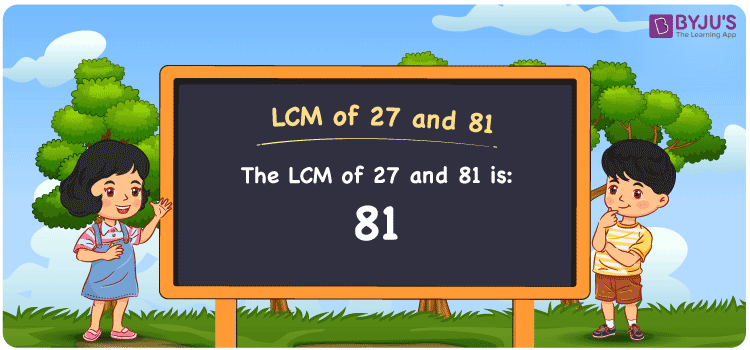# LCM of 27 and 81

LCM of 27 and 81 is 81. The Least Common Multiple or Lowest Common Multiple always known as LCM is the smallest number that is divisible by the given numbers. In the given set of numbers 27 and 81, 81 is the first (least or smallest) number that is common in the set of multiples of 27 and 81. The LCM is also known as LCD, Least Common Divisor. You can use the HCF and LCM to understand the lcm.

## What is LCM of 27 and 81

The Least Common Multiple or Lowest Common Multiple of 27 and 81 is 81.## How to Find LCM of 27 and 81?

LCM of 27 and 81 can be determined using three methods:

• Prime Factorisation
• Division method
• Listing the multiples

### LCM of 27 and 81 Using Prime Factorisation Method

In the Prime Factorisation method, the numbers 27 and 81 can be expressed as;

27 = 3 × 3 × 3

81 = 3 × 3 × 3 × 3

3 × 3 × 3 are the common factors of 27 and 81. This is multiplied by the other factors of 81 to get the LCM.

LCM (27, 81) = 3 × 3 × 3 × 3 = 81

### LCM of 27 and 81 Using Division Method

In the Division Method, the numbers 27 and 81 are divided together by common prime divisors. The division is continued with uncommon prime divisors, if required. The product of common and uncommon prime divisors forms the LCM.

 3 27 81 3 9 27 3 3 9 3 1 3 × 1 1

LCM (27, 81) = 3 × 3 × 3 × 3 = 81

### LCM of 27 and 81 Using Listing the Multiples

By listing all the multiples of 27 and 81 we can identify the LCM. Below is the list of multiples for 27 and 81

 Multiples of 27 Multiples of 81 27 81 54 162 81 243

LCM (27, 81) = 81

## Video Lesson on Applications of LCM## Solved Examples

What is the smallest number that is divisible by both 27 and 81?

Answer: 81 is the smallest number that is divisible by both 27 and 81.

What is the LCM for 3, 9, 27 and 81?

Answer: LCM for 3, 9, 27 and 81 is 81 as 3 and 9 are the factors of both 27 and 81.

## Frequently Asked Questions on LCM of 27 and 81

### What is the LCM of 27 and 81?

The LCM of 27 and 81 is 81.

### Is the LCM of 27 and 81 the same as the Highest Common Factor of 27 and 81?

No. LCM of 27 and 81 is 81 and the Highest Common Factor of 27 and 81 is 27.

### What is the common factor of 27 and 81?

1, 3, 9 and 27 are the common factors of  27 and 81.

### What are the first 3 common multiples of 27 and 81?

The first three common multiples of 27 and 81 are 81, 162, and 243.

### Is the LCM of 9 and 27 same as the LCM of 9 and 42?

No. LCM of 9 and 27 is 27, whereas the LCM of 9 and 42 is 126. They are not the same.